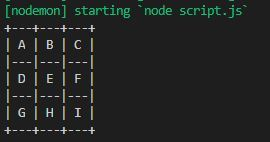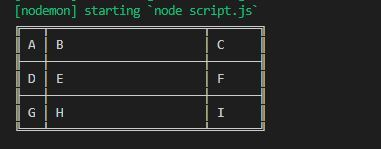Open in App
Not now

# How to display output data in tabular form in Node.js ?

• Last Updated : 01 Jul, 2020

Tables are a combination of rows and columns. Node.js has its own module named as a table that helps in making tables with a wide variety of styles that can be applied to the table such as border styles, colors body style, etc.

Installation of module:

`npm install table`

Syntax:

`table(data, config)`

Parameters:

• data: Data is an Array of array i.e. data to be saved in the table.
• config: Different predefined configuration.

Return Value: A string is returned by the function.

Example 1: Filename: script.js

 `let table = require(``"table"``);``let data, config;`` ` `// Data to be saved in the tables``data = [``  ``[``"A"``, ``"B"``, ``"C"``],          ``  ``[``"D"``, ``"E"``, ``"F"``],``  ``[``"G"``, ``"H"``, ``"I"``],``]`` ` `config = {`` ` `  ``// Predefined styles of table``  ``border: table.getBorderCharacters(``"ramac"``),``}`` ` `let x = table.table(data, config);``console.log(x)`

Step to run this program: Run script.js using the following command:

`node script.js`

Output:Example 2: Filename: script.js

 `let table = require(``"table"``);``let data, config;`` ` `data = [``  ``[``"A"``, ``"B"``, ``"C"``],``  ``[``"D"``, ``"E"``, ``"F"``],``  ``[``"G"``, ``"H"``, ``"I"``],``]`` ` `// Creating column width configuration``config = { ``  ``columns: {``    ``0: {``      ``width: 1   ``// Column 0 of width 1``    ``},``    ``1: {``      ``width: 20  ``// Column 1 of width 20``    ``},``    ``2: {``      ``width: 5   ``// Column 2 of width 5``    ``}``  ``}``};``let x = table.table(data, config);``console.log(x)`

Step to run this program: Run script.js using the following command:

`node script.js`

Output:My Personal Notes arrow_drop_up